Sponsored Link •Weblogs Forum
The Adventures of a Pythonista in Schemeland/16

3 replies on 1 page. Most recent reply: Feb 16, 2009 9:54 PM by Michele Simionato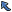Back to Topic List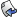Reply to this Topic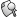Search Forum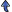Threaded View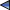Previous Topic Next Topic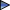Flat View: This topic has 3 replies on 1 page
 Michele Simionato Posts: 222 Nickname: micheles Registered: Jun, 2008
The Adventures of a Pythonista in Schemeland/16 (View in Weblogs)
Posted: Feb 16, 2009 9:54 PMReply
Summary
This episode is a direct continuation of latest issue: it gives example of use of the destructuring bind form let+ introduced there. I also discuss multiple values, unary functions and functions with optional arguments.
 Advertisement# list destructuring versus let-values

There is a feature of Scheme that I never liked, i.e. the fact that functions (more in general continuations) can return multiple values. Multiple values are a relatively late addition to Scheme - they entered in the standard with the R5RS report - and there has always been some opposition against them (see for instance this old post by Jeffrey Susskind). I personally see multiple values as a wart of Scheme, a useless complication motivated by premature optimization concerns.

It is possible to define functions returning multiple values as follows:

```> (define (return-three-values)
(values 1 2 3))
> (return-three-values)
1
2
3
```

In order to receive the values a special syntax is needed, and you cannot do things like the following:

```> (apply + (return-three-values))
Unhandled exception
Condition components:
1. &assertion
2. &who: apply
3. &message: "incorrect number of values returned to single value context"
4. &irritants: ((1 2 3))
```

Instead, you are forced to use let-values or other constructs which are able to accept values:

```> (let-values (((x y z) (return-three-values))) (+ x y z))
6
```

In this series I will never use functions returning multiple values, except the ones in the Scheme standard library (this is why I am forced to talk about let-values). Instead of using multiple values, I will return a list of values and I will destructure it with let+. For instance, I will write

```> (let+ ((a b) (list 1 2)) (cons a b))
(1 . 2)
```

```> (let-values (((a b) (values 1 2))) (cons a b))
(1 . 2)
```

let+ is more elegant and more general than let-values: everything let-values can do, let+ can do too. let+ can even faster - in some implementations and in some cases. Here is a benchmark in Ikarus Scheme:

```running stats for (repeat 10000000 (let-values (((x y z) (values 1 2 3))) 'dummy)):
no collections
276 ms elapsed cpu time, including 0 ms collecting
277 ms elapsed real time, including 0 ms collecting
0 bytes allocated

running stats for (repeat 10000000 (let+ ((x y z) (list 1 2 3)) 'dummy)):
58 collections
211 ms elapsed cpu time, including 42 ms collecting
213 ms elapsed real time, including 43 ms collecting
240016384 bytes allocated
```

As you see, let+ takes only 211 ms to unpack a list of three elements ten million times; let-values would take 276 ms instead. On the other hand, let+ involves garbage collection (in our example 24 bytes are allocate per cycle, and thats means 240 million of bytes) and depending on the situations and the implementation this may cause a serious slowdown. You may find much better benchmarks than mine in this thread on comp.lang.scheme and you will see that you can get any kind of results. let-values seems to be slow in Ikarus and in Ypsilon with the default optimization options; it can be faster in other implementations, or in the same implementations with different options or in different releases.

However, those are implementation details. The important thing in my view is the conceptual relevance of a language construct. Conceptually I think the introduction of multiple values in Scheme was a mistake, since it added nothing that could not be done better with containers. I think functions should always return a single value, possibly a composite one (a list, a vector, or anything else). Actually, I am even more radical than that and I think that functions should take a single value, as in SML and Haskell.

# Variadic functions from unary functions

If you have a minimalistic mindset (as I have) you will recognize that multiple argument functions are useless since they can be implemented as unary functions performing destructuring. Here is a simple implementation of the idea:

```(def-syntax (fn (arg ... . rest) body body* ...)
#'(lambda (x)
(let+ ((arg ... . rest) x)
(begin body body* ...))))

(def-syntax (define/fn (name arg ... . rest) body body* ...)
#'(define name (fn (arg ... . rest) body body* ...)))
```

Here are a few examples of usage:

```> (define/fn (double x) (* 2 x))
> (double '(1))
2

> (define/fn (sum . vals) (apply + vals))
> (sum '(1 2 3))
6

> (define/fn (sum-2D (x1 y1) (x2 y2)) (list (+ x1 x2) (+ y1 y2)))
> (sum-2D '((1 2)(3 4)))
(4 6)
```

All the functions defined via define/fn take a single argument, a list, which is then destructured according to the declared structure. double expects a list with a single element named x; sum expects a list with a variable number of elements val; sum-2D expects a two-element lists made of two-element lists named (x1 y1) and (x2 y2) respectively. You can easily imagine more complex examples with deeply nested lists.It is interesting to notice that Python has the list destructuring/tuple unpacking functionality built-in:

```>>> def sum2D((x1, y1), (x2, y2)):
...     return x1 + x2, y1 + y2
...
>>> sum2D((1,2),(3,4))
(4, 6)
```

This is valid Python code in all versions of Python before Python 3.0. However, in Python 3X this functionality has been removed for lack of use (sic).

The advantage of unary functions is that they are easier to compose, and many functional patterns (including currying described in episode #14) becomes possible. However, Scheme is not ML or Haskell, so let us accept functions with multiple arguments and let us take advantage of them to implement optional arguments. This is the subject of the next paragraph.

# Further examples of destructuring: opt-lambda

A weekness of standard Scheme is the lack of functions with default arguments and keyword arguments. In practice, this is a minor weakness since there many libraries implementing the functionality, although in different ways, as usual. I recommend you to look at SRFI-88 and SRFI-89 for more context. Here I will implement equivalent functionality from scratch, as yet another exercise to show the power of let+. Let me start from an example, to make clear the intended functionality. Let me define a function f with optional arguments as follows:

```(define/opt (f x y (opt (u 1) (v 2)) . rest)
(printf "Required: ~a Optional: ~a Other: ~a\n"
(list x y) (list u v) rest))
```

Here x and y are required arguments, u and v are optional arguments and rest are variadic arguments. If you do not provide an optional argument, its default value is be used instead, and f behaves as follows:

```> (f 'a 'b 'c 'd 'e 'f)
Required: (a b) Optional: (c d) Other: (e f)
> (f 'a 'b 'c)
Required: (a b) Optional: (c 2) Other: ()
> (f 'a 'b)
Required: (a b) Optional: (1 2) Other: ()
> (f 'a)
Unhandled exception
Condition components:
1. &assertion
2. &who: apply
3. &message: "incorrect number of arguments"
4. &irritants: (#<procedure f> 1)
```

It is clear that in order to implement the functionality the trick is to override the defaults of the optional argument with the passed arguments, if any. To this aim we will need the following helper function:

```;;(override-with '(a b) '(1 2 3)) => '(a b 3)
(define (override-with winner loser)
(let ((w (length winner)) (l (length loser)))
(if (>= w l)
winner ; winner completely overrides loser
(append winner (list-tail loser w)))))
```

(we introduced the list-tail function in episode #13). At this point it is easy to define an opt-lambda macro doing the job:

```(def-syntax opt-lambda
(syntax-match (opt)
(sub (opt-lambda (r1 ... (opt (o1 d1) ...) . rest) body1 body2 ...)
#'(lambda (r1 ... . args)
(let+ ((o1 ... . rest) (override-with args (list d1 ...)))
(begin body1 body2 ...))))))
```

define/opt is just sugar over opt-lambda:

```(def-syntax (define/opt (name . args) body1 body2 ...)
#'(define name (opt-lambda args body1 body2 ...)))
```

I should notice that strictly speaking you do not need a full restructuring form to implement opt-lambda: since override-with-args returns a flat list, a form which is able to destructure flat list is enough. You could implement it easily enough:

```(def-syntax (let- (name ... . rest) lst expr)
#'(apply (lambda (name ... . rest) expr) lst))
```

However let+ subsumes the functionality of let- and I do not see the point of introducing yet another binding form, except for sake of the exercise. Strangely enough, let- looks even slower than let+ in Ikarus:

```running stats for (repeat 10000000 (let- (x y z) (list 1 2 3) 'dummy)):
58 collections
324 ms elapsed cpu time, including 16 ms collecting
324 ms elapsed real time, including 22 ms collecting
240004096 bytes allocated
```

But please don't trust benchmarks! ;)

 John Cowan Posts: 36 Nickname: johnwcowan Registered: Jul, 2006
Re: The Adventures of a Pythonista in Schemeland/16 Posted: Feb 16, 2009 10:49 PMReply
You're quite right to say that multiple values are the dual of multiple arguments, and if you disapprove of the latter, it's not surprising that you disapprove of the former. But if you accept multiple arguments and CPS, then multiple values are just passing multiple arguments to the continuation.

The two main irritants around multiple values are that unlike arguments there is no way to name them individually within a function, and <i>a fortiori</i> there is no way for a compiler to determine whether a function returns a fixed number of values or even how many it returns (short of interprocedural analysis). That winds up creating run-time inefficiency even in CPS-converting compilers like Chicken.

Multiple values are old in programming: call-by-result, and for some purposes call-by-reference, are equivalent to multiple values. Ada's <code>out</code> and <code>in out</code> modes are a fairly modern example.

 Elizabeth Wiethoff Posts: 89 Nickname: ewiethoff Registered: Mar, 2005
Re: The Adventures of a Pythonista in Schemeland/16 Posted: Feb 17, 2009 11:59 AMReply
> I think functions should always return a single value, possibly a composite one (a list, a vector, or anything else).
`>>> def return_three_values(): return 1, 2, 3... >>> return_three_values()(1, 2, 3)`

Agree. It's nice that this Python function takes what appear to be three return values and returns them as a single tuple.

What I think is really loony is the ability for a Perl function to return either an array or a scalar depending upon what the receiver expects. But perhaps I'm getting off topic.

 william 141 Posts: 1 Nickname: william141 Registered: Feb, 2009
For More Detail visit here Posted: Feb 26, 2009 7:59 PMReply
For More Detail visit herePrevious Topic Next TopicWeb Artima.com